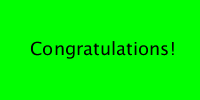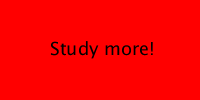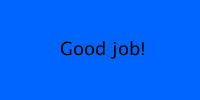Tutorials / p5.js Tutorials / If Statements

# If Statements

tutorial p5.js javascript if-statements

Now you know how to call functions, use variables, and create variables and creating functions.

Most values and variables you’ve seen so far have been numbers. This tutorial introduces the idea of a boolean, which can only hold two possible values: `true` or `false`. This tutorial also introduces if statements, which allow you to perform different actions depending on the value of a boolean.

# Booleans

Remember that to create a variable, you use the `let` keyword to give your variable a name and a value:

``````let catLives = 9;
let accountBalance = -123.45;
let message = 'Happy Coding!';
``````

You can create a boolean variable the same way, but it can only hold two possible values: `true` or `false`.

``````let isCodingFun = true;
``````

This might not seem very useful yet, but it will become more handy in a couple paragraphs. Keep reading!

# Relational Operators

Boolean variables wouldn’t be very useful if you had to decide their values ahead of time. Instead, you can obtain boolean values through relational operators. You might have called these inequalities in algebra class, where you compared two sides with symbols like `<` less than, `>` greater than, or `==` equal to. Here’s an example:

``````let score = 95;
let isGradeA = score >= 90;
``````

This code creates a variable named `score` and sets it equal to `95`. It then creates a boolean variable named `isGradeA` and sets it equal to the result of the inequality `score >= 90`. In this case, the inequality is `true` because `95` is greater than `90`. So at the end of this code, `isGradeA` is holding the boolean value of `true`.

If that line of code is confusing, try reading the right side first. First you take the inequality `score >= 90` and get a boolean value from it (in this case, `true`), and then you point the `isGradeA` variable to that value.

# Boolean Operators

Similar to how you can add two numbers using the `+` operator to get a third number, or subtract them using the `-` operator, you can also operate on two boolean values to get a third boolean value.

## And

You can combine two boolean values using the and operator, which looks like two ampersands: `&&`. The and operator evaluates to `true` whenever the two boolean values on either side of it are also `true`.

``````let canSwim = true;
let canFly = true;
let isDuck = canSwim && canFly;
``````

The `isDuck` variable will only be `true` when both `canSwim` and `canFly` are also true. If either one of them is false, then `isDuck` will also be false.

Again, it might make more sense to read the right side first. First the code evaluates the `&&` operator, which creates a boolean value of `true`. Then it points the `isDuck` variable to that value.

## Or

The or operator evaluates to true if either of the two boolean values on either side of it is `true`.

To use the or operator, type two pipes `||` (they’re above the enter key, or `shift + \`) between two boolean values:

``````let isTodaySaturday = true;
let isTodaySunday = false;
let isTodayWeekend = isTodaySaturday || isTodaySunday;
``````

The `isTodayWeekend` variables will be `true` if either the `isTodaySaturday` or `isTodaySunday` variables are `true`.

First the code evaluates the `||` operator which creates a boolean value (in this case it’s `true`), and then it points the `isTodayWeekend` variable to that value.

## Not

In addition to operating on two `boolean` values, you can also calculate the opposite of a single boolean value. The opposite of `true` is `false`, and the opposite of `false` is `true`.

This is called the not operator, and you use it by typing an exclamation point `!` before the value you want to switch.

``````let sinks = !canSwim;
let falls = !canFly;
let isTodayWeekday = !isTodayWeekend;
``````

Each line of this code evaluates the `!` operator which creates a new boolean value based on the opposite of whatever follows it, and then it points a boolean variable to that value.

## Combining Operators

You can also combine these operators to form more complicated logic. So you can do things like this:

``````let isMammal = !canSwim && !canFly;
``````

First this code takes the opposite of the `canSwim` and `canFly` variables to create two new boolean values. It then takes those values and feeds them into the `&&` operator, which creates yet another boolean value. Then it points the `isMammal` variable to that value.

(If this bothers you because it doesn’t account for animals like bats, beavers, and dolphins… check out the homework!)

There is a whole field of study devoted to boolean logic, so check that out if it sounds interesting. But for now, keep in mind that boolean values contain `true` or `false`, and you can use operators like `&&`, `||`, and `!` on them.

# If Statements

An `if` statement checks a boolean value and only executes a block of code if that value is `true`.

To write an `if` statement, write the keyword `if`, then inside parentheses `()` insert a boolean value, and then in curly brackets `{}` write the code that should only execute when that value is `true`. That code is called the body of the `if` statement.

Here’s an example that draws a congratulations message, but only if your grade is an A:

This code uses an `if` statement to check whether `isGradeA` is `true`, and if it is, it draws a green background, changes the fill color to black and the text size to `18`, and then writes `'Congratulations!'` on the canvas.

Since `score` is `95` (and `95` is greater than `90`), then you see the congratulations message:If `isGradeA` is `false` (if `score` is less than `90`), then the program doesn’t do anything. Try changing `score` to `85`, and you’ll see a blank canvas:Since `isGradeA` is now `false`, the body of the `if` statement is not executed, and it skips over all of the code inside the body.

## Boolean Expressions

So far, all of the examples have separated the inequality and the `if` statement into two steps: the code first created a boolean variable from an inequality, and then it used that variable in an `if` statement. But you can combine them into a single step:

``````function setup() {
createCanvas(200, 100);
background(200);
}

function draw() {
let score = 95;

if (score >= 90) {
background(0, 255, 0);
fill(0);
textSize(18);
text('Congratulations!', 30, 55);
}
}
``````

This does the exact same thing as the old code, except now the inequality (which evaluates to a boolean value) is inside the `if` statement instead of being split into its own step. Either approach is fine, so you should use whichever style makes the most sense to you.

# Else Statements

An `if` statement executes some code if its boolean value is `true`, and it skips that code if the boolean value is `false`. But what if you want to do one thing if the value is `true` and a different thing if it’s `false`? Sounds like a job for an `else` statement!

To use an `else` statement, type the `else` keyword after an `if` statement, and then inside curly brackets `{}` put the code you want to execute when the `if` statement’s boolean evaluates to `false`:

This code uses an `if` statement to check whether `score` is greater than or equal to `90`. Since `85` is less than `90`, that inequality is `false`, so the code inside the `if` statement is skipped. Instead, the program jumps to the code inside the body of the `else` statement, which draws a red background and displays the “Study more!” message.You can think about the code like this: “If the score is greater than or equal to 90, then display the ‘Congratulations!’ message. Otherwise, display the ‘Study more!’ message instead.”

# Else-If Statements

An `if` statement executes some code if its boolean evaluates to `true`, and an `else` statement executes code if it evaluates to `false`. But what if you want to take different actions depending on multiple cases? This is where `else-if` statements come in handy.

An `else-if` statement is like a mix between an `else` statement and an `if` statement. You put an `else-if` statement after an `if` statement, and if the `if` statement evaluates to `false`, then the `else-if` statement’s boolean is evaluated:

``````function setup() {
createCanvas(200, 100);
fill(0);
textSize(18);
}

function draw() {
let score = 85;

if (score >= 90) {
background(0, 255, 0);
text('Congratulations!', 30, 55);
}
else if (score >= 80) {
background(0, 100, 255);
text("Good job!", 60, 55);
}
}
``````

This code uses an `if` statement to check whether `score` is greater than or equal to `90`. Since `85` is less than `90`, that inequality is `false`, so the code inside the `if` statement is skipped. The program jumps down to the `else-if` statement and checks the boolean expression inside the `else-if` statement. Since `85` is greater than `80`, the inequality is `true`, and the code inside the body of the `else-if` is executed. The code then draws a blue background and and displays the `'Good job!'` message.You can think about the code like this: “If the score is greater than or equal to 90, then display the ‘Congratulations!’ message. Otherwise check whether the score is greater than or equal to 80. If it is, then display the `Good job!’ message instead.”

If `score` was `95`, then only the code inside the body of the `if` statement would run. The code inside the `else-if` statement would be skipped. And if `score` was `75`, then the boolean expressions of both the `if` statement and the `else-if` statement would evaluate to `false`, so neither one of their bodies would be executed.

# If Else-If Else Combinations

You can follow an `if` statement with multiple `else-if` statements, and you can follow an `else-if` statement with an `else` statement. So you could expand your program to detect every grade:

This code uses a series of `if`, `else if` and `else` statements to run this logic:

• Is `score >= 90`?
• If so, show the ‘Congratulations!’ message and stop checking any other conditions.
• If not, keep checking.
• Is `score >= 80`?
• If so, show the ‘Good job!’ message and stop checking any other conditions.
• If not, keep checking.
• Is `score >= 70`?
• If so, show the ‘Just okay.’ message and stop checking any other conditions.
• If not, keep checking.
• Is `score >= 60`?
• If so, show the ‘Not good!’ message and stop checking any other conditions.
• If not, show the ‘Study more!’ message.

Try changing the `score` variable to see the different messages.

## Avoiding Unnecessary Checks

Keep in mind that only one body of code is entered in a series of `if else-if else` statements. As soon as the code enters one of the `if` or `else-if` statements, it stops checking subsequent `else-if` statements.

Look at this line of code, from the above program:

``````else if (score >= 80) {
``````

Notice that the code only checks whether `score >= 80`. But for the grade to be a B, it also has to be `< 90`. Why doesn’t the code also check for that?

The code doesn’t check to make sure that `score < 90`, because it already knows that it is. If `score` was `>= 90`, then the first `if` statement would have been entered, and the code wouldn’t even reach this line.

Think about it this way: first the code checks whether the grade is an A. If it is, then it displays the “Congratulations!” message and doesn’t check any other grades since it already knows the grade is an A. If it’s not an A, then it knows that `score < 90` and it keeps checking other grades.

That’s why the code can check whether the grade is a B by only checking whether `score > 80`. It it is, then it displays the “Good job!” message and doesn’t check against any other grades since it already knows the grade is a B. If it’s not a B, then it knows that `score < 80`, and it continues that pattern for the rest of the program.

# Truthiness

If you try to use a non-boolean value in an `if` statement, then the code uses the “truthiness” of that value to convert it to a boolean. Values like `0`, `''` (empty string), and `undefined` are “falsy” and convert to `false`, and values like `'42'` and `'hello world'` are “truthy” and convert to `true`.

Here’s an example:

This code creates a `volume` variable, and then uses that variable in an `if` statement. Since it’s not a boolean, the code checks its truthiness to convert it to a boolean.

Try changing `volume` to `0` to see what happens!

• Expand the `boolean` example to work for more animals. Write a `showAnimalType` function that takes a set of `boolean` parameters (as many as you want!) and shows a message that explains whether the animal is a mammal, bird, reptile, amphibian, or fish. How would you represent a bat (which can fly but isn’t a bird) or a penguin (which can’t fly but is a bird)? If you want an advanced challenge, do a google search for “animal identification key” for some handy guides.
• Create a program that uses `boolean` logic to determine the outcome of a flowchart like this xkcd comic flowchart. If you want an advanced challenge, try this xkcd advance flowchart!